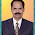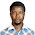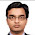## Sunday, May 6, 2018

The effect of wind on a building gets more significant as the height of the building increases. In this post, wind load analysis has been carried out on a 60m tall high rise building using the method described in EN 1991-1-4:2005 (General actions - Wind action). The structure is assumed to be located in an area with a basic wind speed of 40 m/s.

Basic Data
Height of building = 60m
Width of a building = 30m
Structure - Framed building
The structure is located at terrain category II (see Table below)

Basic wind velocity
The fundamental value of the basic wind velocity Vb,0 is the characteristic 10 minute mean wind velocity irrespective of wind direction and time of the year, at 10 m above ground level in open-country terrain with low vegetation such as grass, and with isolated obstacles with separations of at least 20 obstacle heights.

The basic wind velocity Vb,0 is calculated from;
Vb = Cdir . Cseason .Vb,0

Where:
Vb is the basic wind velocity defined as a function of wind direction and time of the year at 10m above the ground of terrain category II
Vb,0 is the fundamental value of the basic wind velocity
Cdir is the directional factor (defined in the National Annex, but recommended value is 1.0)
Cseason is the season factor (defined in the National Annex, but recommended value is 1.0)

For the area and location of the building that we are considering;
Basic wind velocity Vb,0 = 40 m/s
Vb = Cdir. Cseason.Vb,0 = 1.0 × 1.0 × 40 = 40 m/s

Mean Wind
The mean wind velocity Vm(z) at a height z above the terrain depends on the terrain roughness and orography, and on the basic wind velocity, Vb, and should be determined using the expression below;

Vm(z) = cr(z). co(z).Vb

Where;
cr(z) is the roughness factor (defined below)
co(z) is the orography factor often taken as 1.0

The terrain roughness factor accounts for the variability of the mean wind velocity at the site of the structure due to the height above the ground level and the ground roughness of the terrain upwind of the structure in the wind direction considered. Terrain categories and parameters are shown in Table 2.0.

cr(z)  = kr. In (z/z0) for zmin ≤ z ≤ zmax
cr(z) = cr.(zmin) for z ≤ zmin

Where:
Z0 is the roughness length
kr is the terrain factor depending on the roughness length Z0 calculated using;

kr = 0.19 (Z0/Z0,II)0.07

Where:
Z0,II = 0.05m (terrain category II)
Zmin is the minimum height = 2 m
z = 60 m
Zmax is to be taken as 200 m
Kr = 0.19 (0.05/0.05)0.07 = 0.19
cr(60) = kr.In (z/z0) = 0.19 × In(60/0.05) = 1.347

Therefore;
Vm(60) = cr(z). co(z).Vb = 1.347 × 1.0 × 40 = 53.88 m/s

Wind turbulence
The turbulence intensity Iv(z) at height z is defined as the standard deviation of the turbulence divided by the mean wind velocity. The recommended rules for the determination of Iv(z) are given in the expressions below;

Iv(z) = σv/Vm = kl/(c0(z).In (z/z0)) for zmin ≤ z ≤ zmax
Iv(z) = Iv.(zmin) for z ≤ zmin

Where:
kl is the turbulence factor of which the value is provided in the National Annex but the recommended value is 1.0
Co is the orography factor described above
Z0 is the roughness length described above.

For the building that we are considering, the wind turbulence factor at 60m above the ground level;

Iv(60) = σv/Vm = k1/[c0(z).In(z/z0)] = 1/[1 × In(60/0.05)] = 0.141

Peak Velocity Pressure
The peak velocity pressure qp(z) at height z is given by the expression below;

qp(z) = [1 + 7.Iv(z)] 1/2.ρ.Vm2(z) = ce(z).qb

Where:
ρ is the air density, which depends on the altitude, temperature, and barometric pressure tobe expected in the region during wind storms (recommended value is 1.25kg/m3)

ce(z) is the exposure factor given by;
ce(z) = qp(z)/qb
qb is the basic velocity pressure given by; qb = 0.5.ρ.Vb2

qp(60m) = [1 + 7(0.141)] × 0.5 × 1.25 × 53.882 = 3605.23 N/m2

Therefore, qp(60m) = 3.605 kN/m2

External Pressure Coefficients
From Table 7.1 of EN 1991-1-4:2005 (E)
For the building, taking the height to width ratio h/d = 2.0
Pressure coefficient for windward side = +0.8
Pressure coefficient for leeward side = –0.6
The net pressure coefficient Cpe10 = +0.8 – (–0.6) = 1.4
The net external surface pressure on the structure = qp(z) Cpe10 = 3.6057 × 1.4 = 5.05 kN/m2
Therefore, we = 5.05 kN/m2

1.I want to request for PDf file on tall buildings, sodekeaugustine3@gmail.com Thanks

2.Kindly send me a pdf on murageantony24@gmail.com

3.stefano.barone.87@gmail.com

4.Could I kindly have the pdf to this adress giuseppemartino.erbi@gmail.com? Thank you so much. I am following all your fabulous articles. Thank for share them. Kind Regards.

5.maazina.khan@gmail.com

6.Is it possible I get the pdf file on tall buildings sir? bspcltd@yahoo.com
Thank you

7.8.From these calculations, it is not clear that the wind profile varies with height....or the requirements that lead to your assumptions.

9.Please arrange to mail pdf file in my email id:- kishor_bsnl@yahoo.com

10.Please, kindly send this pdf format to email: kayodeoni@aol.com. Thanks and God bless

11.Please provide the PDF file... simplyajaylamba@gmail.com

12.